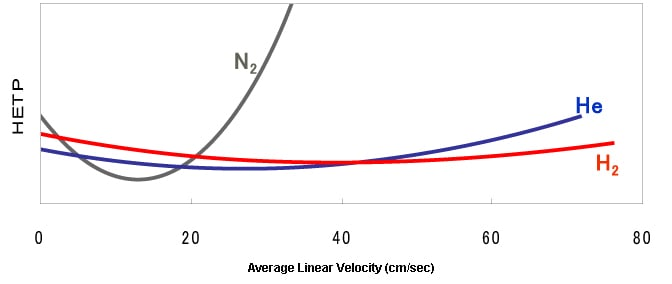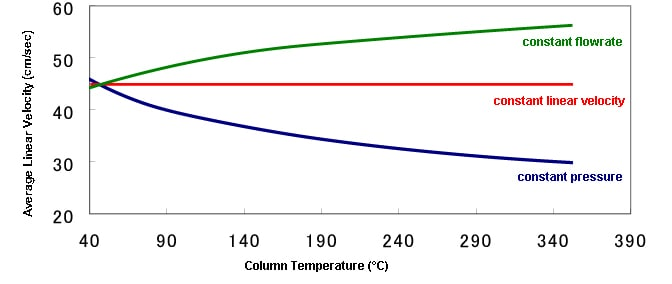# 3. Carrier Gas

### 3.1. Carrier Gas Types

Carrier gas is an inert gas used to carry samples. Helium (He), nitrogen (N2), hydrogen (H2), and argon (Ar) are often used.
Helium and nitrogen are most commonly used and the use of helium is desirable when using a capillary column.

Helium Although expensive, it is safe and has a relatively wide optimum linear velocity range. Although it is safe and its cost is reasonable, it has disadvantages such as a narrow optimum linear velocity range and a low optimum linear velocity that requires more analysis time.

As carrier gas constantly flows into the detector, high-purity gas of at least 99.995 % needs to be used. (The use of high-purity gas reduces baseline noise.)

Relationships between Column Efficiency (HETP), Carrier Gas Types, and Linear Velocity (Conceptual Diagram)*HETP: Height equivalent to a theoretical plate (parameter that indicates the separation efficiency of a column)
Average linear velocity: Average value of the speed of a carrier gas flowing in a column

The column efficiency varies in accordance with the combination of the type of carrier gas used and the analysis conditions (linear velocity).
When using a carrier gas, a suitable linear velocity needs to be specified based on the relationships between the column efficiency (HETP), carrier gas type, and linear velocity shown in the figure above. In conventional analysis using a capillary column, around 30 cm/sec of linear velocity is used because helium is often used as the carrier gas.

### 3.2. Carrier Gas Control Methods

For controlling the carrier gas, there are methods to control the pressure, column flowrate, and linear velocity.
(The method to control the linear velocity is patented by Shimadzu Corporation.)
In most analyses, one of these parameters is controlled at a certain value during the analysis. However, programmed control, such as increasing the pressure during an analysis, can also be used.

Pressure Control Column Flowrate Control Linear Velocity Control
The pressure at the injection port is controlled to match the setting. The flowrate of the carrier gas inside the column is controlled to match the setting. The average linear velocity of the carrier gas inside the column is controlled to match the setting.
Generally used for capillary analysis. Used when injecting the entire amount using a packed or wide-bore column. Can only be used on GC system units that can be controlled electronically.
The pressure fluctuates while the temperature increases. The flow rate fluctuates as the column resistance changes. The pressure is adjusted in accordance with the changes in the column resistance.

In most analyses, either the pressure, column flowrate, or linear velocity is maintained at a certain value using the control methods listed above.

Changes in the Linear Velocity in Different Control Methods (During Programmed Temperature Analysis)0.25mmID × 30m , df=0.25µm
He：150kPa, 2.32mL/min, 45.1cm/sec

When performing programmed temperature analysis, the linear velocity fluctuates as the column temperature increases. This occurs because the diffusion of the carrier gas flowing in the column changes with increases in temperature.
Since the column efficiency (HETP) depends on the linear velocity as described above, fluctuation in the linear velocity during analysis causes fluctuation in the column efficiency. Therefore, it can be said that control of linear velocity is suitable in order to maintain the column efficiency constantly at the same level during analysis.